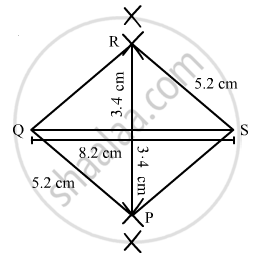Share

# Construct a Parallelogram Pqrs Such that Pq = 5.2 Cm, Pr = 6.8 Cm and Qs = 8.2 Cm. - Mathematics

Course
ConceptConstructing a Quadrilateral When the Lengths of Four Sides and a Diagonal Are Given

#### Question

Construct a parallelogram PQRS such that PQ = 5.2 cm, PR = 6.8 cm and QS = 8.2 cm.

#### SolutionIn a parallelogram opposite sides are equal . Thus, we have to construct a quadrilateral PQRS in which

PQ = 5 . 2 cm, PR = 6 . 8 cm and QS = 8 . 2 cm .

Steps of construction:

Step I: Draw QS = 8 . 2 cm

Step II: With Q as the centre and radius 5 . 2 cm, draw an arc .

Step III: With S as the centre and radius 5 . 2 cm, draw an arc to intersect the arc drawn in Step II at C .

Step IV: With P as the centre and radius 6 . 8 cm .

Step V: With Q as the centre and radius 5 . 2 cm, draw an arc to intersect the arc drawn in Step IV at A .

Step VI: Join QR, QP, PS and SR .